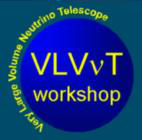#VLVnT08

Apr 22 – 24, 2008
Palais Neptune Conference Center, Toulon
Europe/Paris timezone

## Flavor Distribution of UHE Neutrino Oscillations at Neutrino Telescopes

Not scheduled
20m
Palais Neptune Conference Center, Toulon

#### Palais Neptune Conference Center, Toulon

Palais des Congrès Neptune Place de Besagne Centre Commercial Mayol
Contributed talk Plenary Session

### Speaker

Prof. Zhi-zhong Xing (Institute of High Energy, Chinese Academy of Sciences)

### Description

If the ultrahigh-energy (UHE) neutrino fluxes produced from a distant astrophysical source can be measured at a km^3-size neutrino telescope, they will provide a promising way to help determine the flavor mixing pattern of three active neutrinos. Considering the conventional UHE neutrino source with the flavor ratio \phi_e : \phi_\mu : \phi_\tau = 1 : 2 : 0, I will show that \phi_e : \phi_\mu : \phi_\tau = (1 -2 \Delta) : (1 +\Delta) : (1 +\Delta) holds at the detector of a neutrino telescope, where \Delta characterizes the effect of \mu-\tau symmetry breaking (i.e., \theta_{13} \neq 0 and \theta_{23} \neq \pi/4). Current experimental data yield -0.1 \leq \Delta \leq +0.1. Furthermore, I will propose a parametrization for the flavor composition of UHE neutrino fluxes produced from arbitrary astrophysical sources: \phi_e : \phi_\mu : \phi_\tau = \sin^2 \xi \cos^2 \zeta : \cos^2 \xi \cos^2 \zeta : \sin^2 \zeta. I show that it is possible to determine or constrain \xi and \zeta by observing two independent neutrino flux ratios at neutrino telescopes, provided three neutrino mixing angles and the Dirac CP-violating phase have been well measured in neutrino oscillations. Any deviation of \zeta from zero will signify the existence of cosmic \nu^{}_\tau and \overline{\nu}^{}_\tau neutrinos at the source, and an accurate value of \xi can be used to test both the conventional mechanism and the postulated scenarios for cosmic neutrino production.

### Primary author

Prof. Zhi-zhong Xing (Institute of High Energy, Chinese Academy of Sciences)

### Presentation materials

 Toulon.pdf Toulon.zip Xing-final.pdf Xing-final.zip